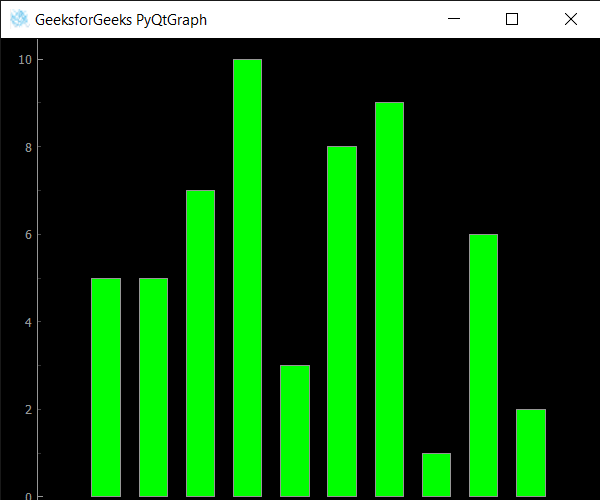# PyQtGraph – Getting Data of Bar Graph

In this article we will see how we can get data of the bar graph in the PyQtGraph module. PyQtGraph is a graphics and user interface library for Python that provides functionality commonly required in designing and science applications. Its primary goals are to provide fast, interactive graphics for displaying data (plots, video, etc.) and second is to provide tools to aid in rapid application development (for example, property trees such as used in Qt Designer).A bar chart or bar graph is a chart or graph that presents categorical data with rectangular bars with heights or lengths proportional to the values that they represent. The bars can be plotted vertically or horizontally. A vertical bar chart is sometimes called a column chart. Bar graph uses two data from two list i.e for x-axis and for the height of the bars.

We can create a plot window and bar graph with the help of commands given below

```# creating a pyqtgraph plot window
window = pg.plot()

# creating a bar graph of green color
bargraph = pg.BarGraphItem(x=x, height=y1, width=0.6, brush='g')
```

In order to do this we use `getData` method with the bar graph object

Syntax : bargraph.getData()

Argument : It takes no argument

Return : It returns tuple

Below is the implementation

 `# importing QtGui to use QIcon ` `from` `PyQt5.QtGui ``import` `*` `from` `PyQt5.QtCore ``import` `Qt  ` ` `  `# importing pyqtgraph as pg ` `import` `pyqtgraph as pg ` ` `  `# importing QtCore and QtGui from the pyqtgraph module ` `from` `pyqtgraph.Qt ``import` `QtCore, QtGui ` ` `  `# importing numpy as np ` `import` `numpy as np ` ` `  `import` `time ` ` `  `# creating a pyqtgraph plot window ` `window ``=` `pg.plot() ` ` `  `# icon for plot window ` `icon ``=` `QIcon(``"logo.png"``) ` ` `  `# setting icon to the plot window ` `window.setWindowIcon(icon) ` ` `  `# setting window geometry ` `# left = 100, top = 100 ` `# width = 600, height = 500 ` `window.setGeometry(``100``, ``100``, ``600``, ``500``) ` ` `  `# title for the plot window ` `title ``=` `"GeeksforGeeks PyQtGraph"` ` `  `# setting window title to plot window ` `window.setWindowTitle(title) ` ` `  `# create list for y-axis ` `y1 ``=` `[``5``, ``5``, ``7``, ``10``, ``3``, ``8``, ``9``, ``1``, ``6``, ``2``] ` ` `  `# create horizontal list i.e x-axis ` `x ``=` `[``1``, ``2``, ``3``, ``4``, ``5``, ``6``, ``7``, ``8``, ``9``, ``10``] ` ` `  `# create pyqt5graph bar graph item  ` `# with width = 0.6 ` `# with bar colors = green ` `bargraph ``=` `pg.BarGraphItem(x ``=` `x, height ``=` `y1, width ``=` `0.6``, brush ``=``'g'``) ` ` `  `# add item to plot window ` `# adding bargraph item to the window ` `window.addItem(bargraph) ` ` `  ` `  `# getting data of the bar graph ` `value ``=` `bargraph.getData() ` ` `  `# printing the value ` `print``(``"Data  : "` `+` `str``(value)) ` ` `  ` `  `# main method ` `if` `__name__ ``=``=` `'__main__'``: ` `     `  `    ``# importing system ` `    ``import` `sys ` `     `  `    ``# Start Qt event loop unless running in interactive mode or using  ` `    ``if` `(sys.flags.interactive !``=` `1``) ``or` `not` `hasattr``(QtCore, ``'PYQT_VERSION'``): ` `        ``QtGui.QApplication.instance().exec_() ` `        `

Output :```Data  : ([1, 2, 3, 4, 5, 6, 7, 8, 9, 10], [5, 5, 7, 10, 3, 8, 9, 1, 6, 2])
```

Whether you're preparing for your first job interview or aiming to upskill in this ever-evolving tech landscape, GeeksforGeeks Courses are your key to success. We provide top-quality content at affordable prices, all geared towards accelerating your growth in a time-bound manner. Join the millions we've already empowered, and we're here to do the same for you. Don't miss out - check it out now!

Previous
Next